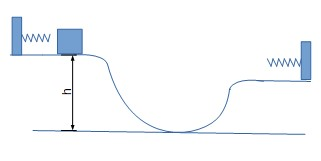# The figure shows a roller coaster, initially the 200 kg car is pushed 3 m against a spring with...

## Question:

The figure shows a roller coaster, initially the 200 kg car is pushed 3 m against a spring with spring constant of 2000 N/m, and is a height h above the lowest point on the track. When released, it descends along the track, and at the lowest point it is observed to travel at 15 m/s

(a) Find the height h.

(b) At the end of the ride, the car is stopped by a spring with a spring constant of 1440 N/m at the end of the track. Compressing it 4.8 m before coming to a complete stop. The end of the track is lower than the initial segment of the track as shown. How much lower is it?## Energy Conservation:

This problem is based on the energy balance equation which is applied for conservative forces. The work is done by gravity, the work stored and released from the spring and the continuously changing kinetic energy of a body is all interchanging from one to another. There is no creation of energy nor destruction. The total mechanical energy at any point in the space will remain constant. The following formula will be applied:

{eq}\begin{align} \frac{k_1 x_1^2} {2}+ \frac{mv_1^2} {2}+mgh_1 &= \frac{k_2 x_2^2} {2}+ \frac{mv_2^2} {2}+mgh_2 \end{align} {/eq}

where,

g is the constant of gravity,

v is the velocity of the car,

x is the spring compression length,

h is the height of the roller coaster above the ground,

subscripts 1 and 2 indicate the initial and the final state respectively.

(a)

We will apply the energy balance equation between the initial position and the lowest position.

{eq}\begin{align} m&=200 \rm{kg}\\ k&=2000 N/m\\ v_1&=0\\ v_2&=15 \rm{m/s}\\ x_1 &=3 \rm{m}\\ h_1 &=h\\ h_2 &=0\\ \frac{k x_1^2} {2}+ \frac{mv_1^2} {2}+mgh_1 &= \frac{mv_2^2} {2}+mgh_2 & \left[\text{This is the energy balance equation } \right]\\ \Rightarrow \frac{(2000) (3)^2} {2}+ \frac{(200) (0) ^2} {2}+(200)(9.81) h &= \frac{(200) (15)^2} {2}+(200)(9.81) (0) \\ \Rightarrow (200)(9.81) h &= \frac{(200) (15)^2} {2} - \frac{(2000) (3)^2} {2} \\ \Rightarrow (200)(9.81) h &= 13500\\ \Rightarrow h &= 6.88\rm{m } \\ \end{align} {/eq}

Therefore, the height is:

{eq}\displaystyle \boxed{\color{blue} { h = 6.88\rm{m } }} {/eq}

(b)

Now, we will apply the energy balance equation between the initial position and the final position.

{eq}\begin{align} m&=200 \rm{kg}\\ k_1&=2000 N/m\\ k_2&=1440 N/m\\ v_1&=0\\ v_2&=0 \rm{m/s}\\ x_1 &=3 \rm{m}\\ x_2 &=4.8 \rm{m}\\ \frac{k_1 x_1^2} {2}+ \frac{mv_1^2} {2}+mgh_1 &= \frac{k_2 x_2^2} {2}+ \frac{mv_2^2} {2}+mgh_2 & \left[\text{This is the energy balance equation between initial and final positions } \right]\\ \Rightarrow \frac{(2000) (3)^2} {2}+\frac{(200) (0) ^2} {2}+(200)(9.81) h_1 &=\frac{(1440) (4.8)^2} {2}+\frac{(200) (0) ^2} {2}+(200)(9.81) h_2\\ \Rightarrow \frac{(2000) (3)^2} {2} +(200)(9.81) h_1 &=\frac{(1440) (4.8)^2} {2}+(200)(9.81) h_2\\ \Rightarrow (200)(9.81) h_1- (200)(9.81) h_2 &=\frac{(1440) (4.8)^2} {2} - \frac{(2000) (3)^2} {2} \\ \Rightarrow (200)(9.81) (h_1- h_2) &= \frac{(1440) (4.8)^2} {2} - \frac{(2000) (3)^2} {2} \\ \Rightarrow (200)(9.81) \Delta h &= 7588.8\\ \Rightarrow \Delta h &= 3.86788 \rm{m } \\ \end{align} {/eq}

Therefore, the height of the second track is lower than the first track by:

{eq}\displaystyle \boxed{\color{blue} {\Delta h = 3.87 \rm{m } }} {/eq}